Study Materials

# NCERT Solutions for Class 9th Mathematics

Page 1 of 4

## Chapter 7. Triangles

### Exercise 7.1

Chapter 7. Triangles

Exercise 7.1

Q1. In quadrilateral ACBD, AC = AD and AB bisects ∠A (see Fig. 7.16). Show that Δ ABC ≅ Δ ABD.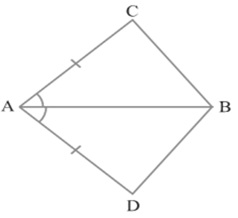Solution:

Given: AC = AD and AB bisects ∠A

To prove:  Δ ABC ≅ Δ ABD.

Proof: In Δ ABC and Δ ABD.

∠CAB = ∠BAD   [AB bisect ∠A]

AB = AB         [Common]

By SAS Congruence Criterion Rule

Δ ABC ≅ Δ ABD

BC = BD [By CPCT]   Proved

Q2. ABCD is a quadrilateral in which AD = BC and ∠ DAB = ∠ CBA (see Fig.7.17). Prove that

(i) Δ ABD ≅ Δ BAC

(ii)   BD = AC

(iii)  ∠ ABD = ∠ BAC

Solution:

Given: ABCD is a quadrilateral in which AD = BC and ∠ DAB = ∠ CBA

To prove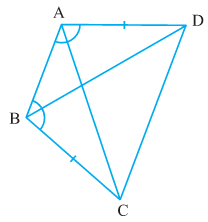(i) Δ ABD ≅ Δ BAC

(ii)   BD = AC

(iii)  ∠ ABD = ∠ BAC

Proof:  (i) In Δ ABD and Δ BAC

∠ DAB = ∠ CBA   [given]

AB = AB         [Common]

By SAS Congruency Criterion Rule

Δ ABD ≅ Δ BAC

(ii)                    BD = AC [CPCT]

(iii)            ∠ ABD = ∠ BAC    [CPCT]

Q3.  AD and BC are equal perpendiculars to a line segment AB (see the given figure). Show that CD bisects AB.

Solution: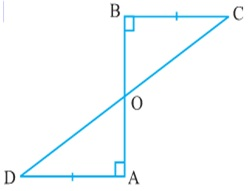Given: AD and BC are equal perpendiculars to a line segment AB.

To prove: CD bisects AB.

Proof:

In ∆BOC and ∆AOD

∠ BOC = ∠AOD (Vertically opposite angles)

∠CBO = ∠DAO (Each 90º)

By AAS Congruence Criterion Rule

∆BOC ≅ ∆AOD

BO = AO (By CPCT)

Hence, CD bisects AB.

Q4. l and m are two parallel lines intersected by another pair of parallel lines p and q (See the given figure). Show that ∆ABC ≅ ∆CDA

Solution: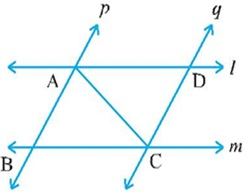Given: l and m are two parallel lines intersected by another pair of   parallel lines p and q.

To prove: ∆ABC ≅ ∆CDA

Proof:

In ∆ABC and ∆CDA,

∠ BAC = ∠DCA (Alternate interior angles, as p || q)

AC = CA (Common)

∠ BCA = ∠DAC (Alternate interior angles, as l || m)

By AAS Congruence Criterion Rule

∆ABC ≅ ∆CDA

Q5. Line l is the bisector of an angle ∠A and B is any point on l. BP and BQ are perpendiculars from B to the arms of a (see the given figure). Show that: (i) ∆APB ≅ ∆AQB (ii) BP = BQ or B is equidistant from the arms of ∠A.

Solution:

Given: Line l is the bisector of an angle ∠A and B is any point on l. BP and BQ are perpendiculars from B to the arms of a.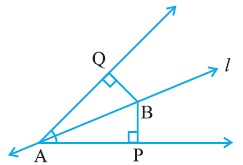To prove:

(i) ∆APB ≅ ∆AQB

(ii) BP = BQ or B is equidistant from the arms of ∠A.

Proof:

In ∆APB and ∆AQB,

∠ APB = ∠AQB (Each 90º)

∠ PAB = ∠QAB (l is the angle bisector of A)

AB = AB (Common)

By AAS Congruence Criterion Rule

∆APB ≅ ∆AQB

BP = BQ    [CPCT]

it can be said that B is equidistant from the A.

Q6. In the given figure, AC = AE, AB = AD and ∠BAD = ∠EAC. Show that BC = DE.

Solution: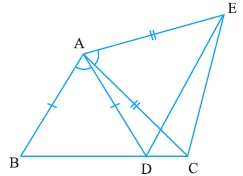To prove: BC = DE.

BAD + DAC = EAC + DAC

BAC = DAE

In ∆BAC and ∆DAE

AC = AE (Given)

∠BAC = ∠DAE (proved above)

By SAS Congruence Criterion Rule

∆BAC ≅ ∆DAE

BC = DE (CPCT)

Q7.  AB is a line segment and P is its mid-point. D and E are points on the same side of AB such that ∠BAD =∠ ABE and ∠EPA = ∠DPB (See the given figure).

Show that:(i) ∆DAP ≅ ∆EBP

Solution:

Given: AB is a line segment and P is its mid-point. D and E are points on the same side Of AB such that ∠BAD =∠ ABE and ∠EPA = ∠DPB.

To prove:

(i) ∆DAP ≅ ∆EBP

Proof:  In ∆ DPA and ∆ EPB

∠EPA = ∠DPB

EPA + DPE = DPB + DPE

∠ DPA = ∠EPB

∠EPA = ∠DPB (Given)

AP =BP (P is the midpoint of AB)

By AAS Congruence Criterion Rule

∆DAP ≅ ∆EBP

Page 1 of 4

Chapter Contents: